# Intermolecular Forces and The Gas Laws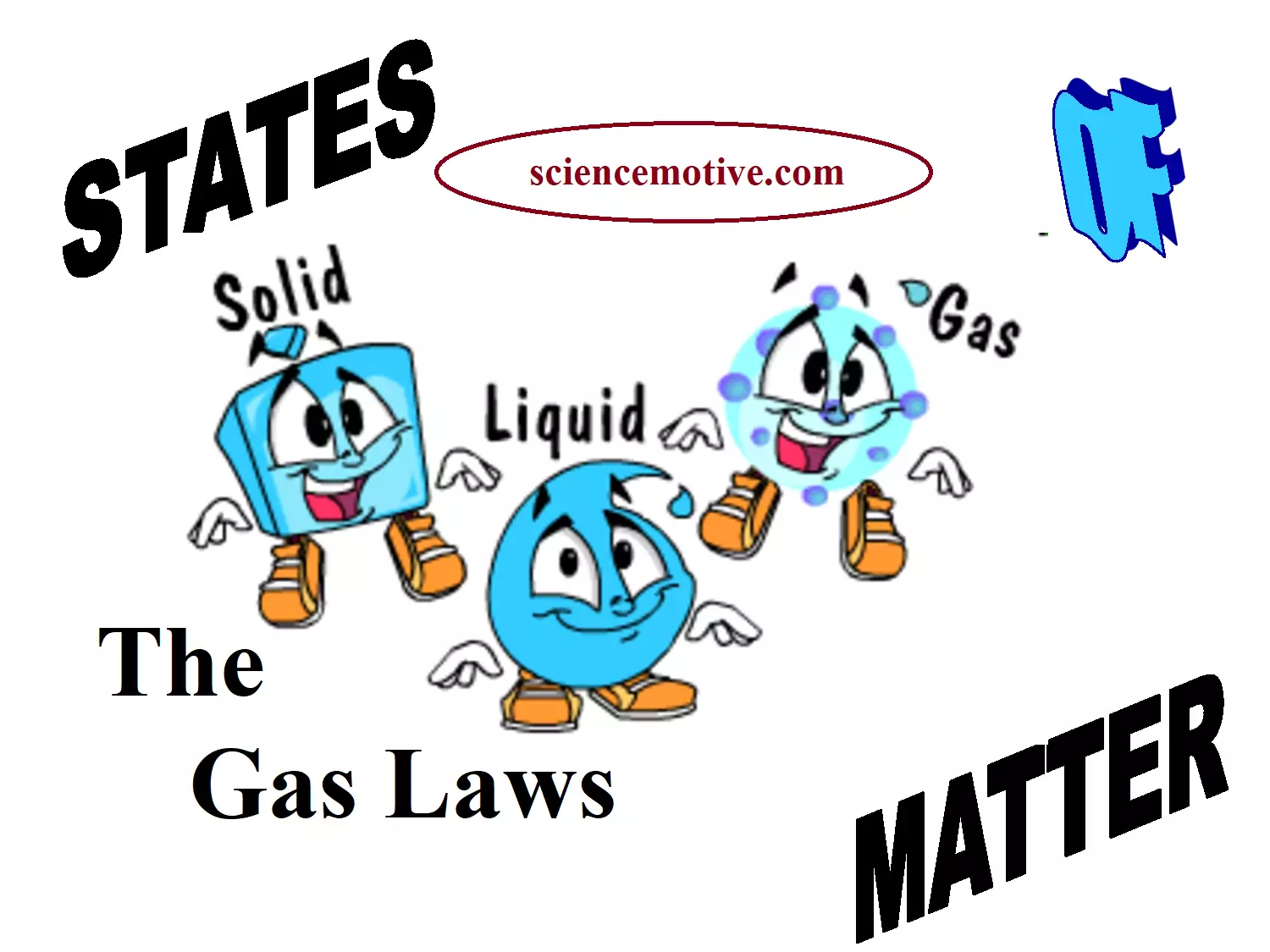Intermolecular Forces and The Gas Laws

Intermolecular Forces and The Gas Laws

Introduction: Matter is anything that occupies space and has a definite mass. Matter mainly exists in three different states – solid, liquid, and gaseous states.

Solids have a definite shape and definite volume. This is because in solids the particles are closely packed and so the intermolecular force of attraction is greater.

Liquids have no definite shape but have a definite volume. In liquids, the intermolecular force of attraction is smaller than that in solids. So, the particles do not have a fixed position.

Gases have no definite shape and volume. Here the particles are far apart and hence they have no force of attraction.

Difference Between three States of Matter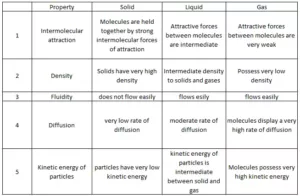Intermolecular Forces: These are the forces of attraction or repulsion between different interacting particles (atoms and molecules).

Attractive intermolecular forces are known as van der Waals forces which include dispersion forces or London forces, dipole-dipole forces, and dipole – induced dipole forces.

Dispersion Forces or London Forces: The interaction which is present between two non-polar molecules. Atoms and non-polar molecules are electrically symmetrical and have no dipole moment. But in an atom, at a particular moment, the nucleus is shifted towards one side and the electrons, to the other side. So, a temporary dipole (momentarily dipole) is created. This results in the development of instantaneous dipole on the adjacent atom for a very short time. These temporary dipoles of atoms attract each other. This force of attraction between temporary dipoles is termed as London forces or dispersion forces.These forces are important only at short distances.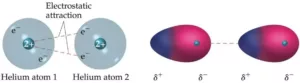Dipole-dipole Forces: The interaction which is present between molecules having permanent dipoles i.e., between polar molecules, for example, NH3, HCl, etc. This interaction is stronger than the London forces but is weaker than ion-ion interaction because only partial charges are involved. The attractive force decreases with the increase of distance between the dipoles.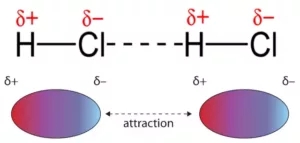Dipole-induced dipole forces: The forces operate between the polar molecule having permanent dipole and the molecule having no permanent dipole. The permanent dipole of the polar molecule induces dipole on the electrically neutral molecule by deforming its electronic cloud. Thus, an induced dipole is developed in the other molecule. The attraction between these molecules is termed as dipole – induced dipole force.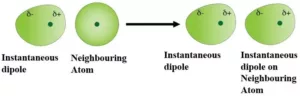Hydrogen Bond: It is a special case of dipole-dipole interaction. When a hydrogen atom is bonded to atoms of highly electronegative elements such as fluorine, oxygen, or nitrogen called a hydrogen bond, the hydrogen atom forms a weak bond with the electronegative atom of the other molecule. This weak bond is called a hydrogen bond.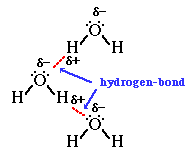Intermolecular Forces and Thermal Energy: Intermolecular force is the attractive force acting between the neighboring molecules. Whereas thermal energy is the measure of the sum of the kinetic energy of the individual molecules and particles. So, the intermolecular force of attraction keeps the particles together while the thermal interactions (kinetic energy) make them move apart.

In gases, the intermolecular forces of attraction are weakest while thermal energy is highest (manifested as the random translatory motion of molecules). In solids, intermolecular forces of attraction are strongest while thermal energy is minimum (only due to vibratory motion about the mean position of constituent particles and no translatory motion at all). In liquids, the two types of energies are intermediate between those of gases and solids.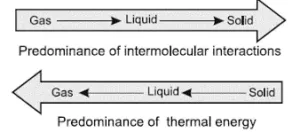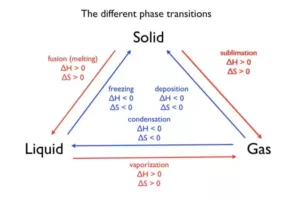The Gaseous State

It is the simplest state of matter. It has the following properties.
(i) Gases are highly compressible
(ii) Gases exert pressure equally in all directions
(iii) Gases have density much lower than the solids and liquids
(iv) Gases do not have definite volume and shape. They assume the volume and shape of the container
(v) Gases mix evenly and completely in all proportions without any mechanical aid.

The Gas Laws

Boyle’s Law (Pressure-Volume Relationship): Chemist and physicist Robert Boyle published the law in 1662. At constant temperature, the pressure of a fixed amount of gas is inversely proportional to its volume.
Mathematically,
P α 1/V
P = k1 × 1/V, where k1 is the proportionality constant.
k1 constant will be dependent on the amount of the gas and temperature of the gas.
Consider a fixed amount of gas at constant temperature T. Let V1 & P1 are its initial volume and pressure respectively. Let the gas undergoes expansion, so that its final volume and pressure becomes V2 and P2.
Then according to Boyle’s law,
P1V1 = P2V2
If we plot graphs between
a). Pressure against Volume:
b). Pressure against 1/Volume: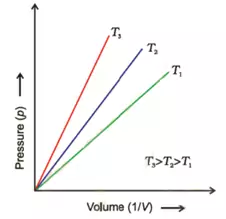c). PV against Pressure: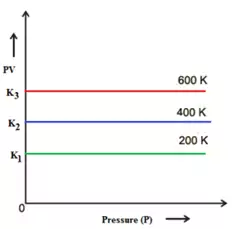These graphs are obtained at constant temperature and are called isotherms.

We know that density = mass/volume
i.e., d = m/V
If we put the value of V in this equation from Boyle’s law equation, we get the relationship, d = (m/k) × p
At constant temperature, the pressure is directly proportional to the density of a fixed mass of the gas.

Boyle’s Law and Human Breathing
Boyle’s law may be applied to explain how people breathe and exhale air. When the diaphragm expands and contracts, lung volume increases, and decreases, changing the air pressure inside of them. The pressure difference between the interior of the lungs and the external air produces either inhalation or exhalation.

Charle’s Law (Volume – Temperature Relationship): The volume of a fixed mass of a gas is directly proportional to absolute temperature at constant pressure. Mathematically, it is written as

 V α T

Or,                       V = k2 x T
Or,                       V/T = k2, a constant
Consider a fixed amount of gas at constant pressure P. Let V1 be its volume at a temperature T1 and V2 be its volume at a temperature T2.
Then according to Charle’s law:

 V1/T1 = V2/T2

If the volume is plotted against temperature at constant pressure, the graph obtained is as follows.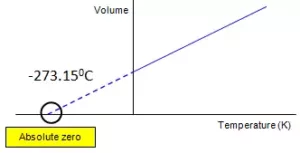Since the graph is obtained at constant pressure, it is called Isobar.
If we extend the graph to the temperature axis (X-axis), the graph will meet at -273.150C. At this temperature, the volume of the gas becomes zero. This lowest hypothetical or imaginary temperature at which gases are supposed to occupy zero volume is called Absolute zero of temperature and the scale of temperature based on absolute zero is called the Absolute scale of temperature. All gases become solid or liquid before reaching this temperature.

Note: 1. All gases obey Charle’s law at very low pressures and high temperatures.

1. Boyle’s and Charles’ law are examples of a limiting law, a law i.e. strictly true only in a certain limit, in this case, p→0.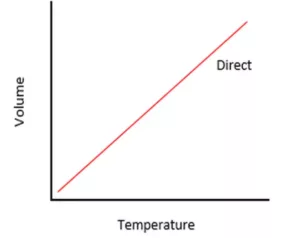Gay Lussac’s law: It states that at constant volume, the pressure of a fixed amount of a gas is directly proportional to the temperature.
Mathematically,

 p α T (V, n constant)

P/T  =  k3 (a constant)

If we plot a graph between the pressure and temperature of a fixed mass of gas at constant volume, the graph obtained is as follows:Applications of Gay-lussac’s law. For the two parts ‘A’ and ‘B’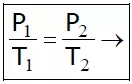the temperature on an absolute scale.

Intermolecular Forces and The Gas Laws

Standard Temperature and Pressure mean 273.15 K (00C) temperature and 1 bar (i.e., exactly 105 Pascal) pressure.

Standard Ambient Temperature and Pressure (SATP), conditions are also used in some scientific works, SATP conditions mean 298.15 k and 1 bar (i.e. exactly 105 Pa) At SATP (1 bar and 298.15 K), the molar volume of an ideal gas is 24.789 L mol-1.

Avogadro’s Hypothesis (Volume – Amount Relationship):
It states that equal volumes of all gases under the same conditions of temperature and pressure contain an equal number of molecules. This means that at constant temperature and pressure, the volume of a gas is directly proportional to its number of moles (n) or molecules (N).
Mathematically, it is written as

 V α n V = k4 n

where k4 is a constant
We know that number of moles (n) = mass in gram(w)/molar mass (M)
i.e., n = w/M
So the above equation becomes:
V = k × w/M
Or,                      M = k × w/V
Or,                      M = k × d
Or,                      M α d
i.e., the density of a gas is directly proportional to its molar mass.

Intermolecular Forces and The Gas Laws